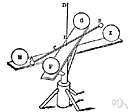# constant of gravitation

Also found in: Thesaurus, Encyclopedia, Wikipedia.
ThesaurusAntonymsRelated WordsSynonymsLegend:
 Noun 1constant of gravitation - (physics) the universal constant relating force to mass and distance in Newton's law of gravitationlaw of gravitation, Newton's law of gravitation - (physics) the law that states any two bodies attract each other with a force that is directly proportional to the product of their masses and inversely proportional to the square of the distance between themnatural philosophy, physics - the science of matter and energy and their interactions; "his favorite subject was physics"constant - a number representing a quantity assumed to have a fixed value in a specified mathematical context; "the velocity of light is a constant"
Based on WordNet 3.0, Farlex clipart collection. © 2003-2012 Princeton University, Farlex Inc.
References in periodicals archive ?
From this point of view, Newton's constant of gravitation defines the corresponding amount of gravitational mass a given attractor node can accumulate.
The lead article in the annual Buyer's Guide of the American Institute of Physics features five pages of values of the fundamental physical constants, such as the speed of light, the Newtonian constant of gravitation, the Planck constant, the fine-structure constant, and energy-unit conversion factors.
And secondly, directly from (2) one can express the value of the Newtonian constant of gravitation (G) exactly as follows:

Site: Follow: Share:
Open / Close